Diffraction

# 30 X-Ray Diffraction

### Learning Objectives

By the end of this section, you will be able to:

• Describe interference and diffraction effects exhibited by X-rays in interaction with atomic-scale structures

Since X-ray photons are very energetic, they have relatively short wavelengths, on the order of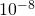m to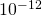m. Thus, typical X-ray photons act like rays when they encounter macroscopic objects, like teeth, and produce sharp shadows. However, since atoms are on the order of 0.1 nm in size, X-rays can be used to detect the location, shape, and size of atoms and molecules. The process is called X-ray diffraction, and it involves the interference of X-rays to produce patterns that can be analyzed for information about the structures that scattered the X-rays.

Perhaps the most famous example of X-ray diffraction is the discovery of the double-helical structure of DNA in 1953 by an international team of scientists working at England’s Cavendish Laboratory—American James Watson, Englishman Francis Crick, and New Zealand-born Maurice Wilkins. Using X-ray diffraction data produced by Rosalind Franklin, they were the first to model the double-helix structure of DNA that is so crucial to life. For this work, Watson, Crick, and Wilkins were awarded the 1962 Nobel Prize in Physiology or Medicine. (There is some debate and controversy over the issue that Rosalind Franklin was not included in the prize, although she died in 1958, before the prize was awarded.)

(Figure) shows a diffraction pattern produced by the scattering of X-rays from a crystal. This process is known as X-ray crystallography because of the information it can yield about crystal structure, and it was the type of data Rosalind Franklin supplied to Watson and Crick for DNA. Not only do X-rays confirm the size and shape of atoms, they give information about the atomic arrangements in materials. For example, more recent research in high-temperature superconductors involves complex materials whose lattice arrangements are crucial to obtaining a superconducting material. These can be studied using X-ray crystallography.

X-ray diffraction from the crystal of a protein (hen egg lysozyme) produced this interference pattern. Analysis of the pattern yields information about the structure of the protein. (credit: “Del45”/Wikimedia Commons)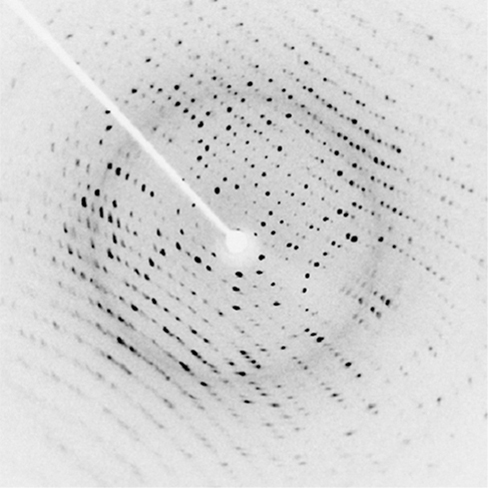Historically, the scattering of X-rays from crystals was used to prove that X-rays are energetic electromagnetic (EM) waves. This was suspected from the time of the discovery of X-rays in 1895, but it was not until 1912 that the German Max von Laue (1879–1960) convinced two of his colleagues to scatter X-rays from crystals. If a diffraction pattern is obtained, he reasoned, then the X-rays must be waves, and their wavelength could be determined. (The spacing of atoms in various crystals was reasonably well known at the time, based on good values for Avogadro’s number.) The experiments were convincing, and the 1914 Nobel Prize in Physics was given to von Laue for his suggestion leading to the proof that X-rays are EM waves. In 1915, the unique father-and-son team of Sir William Henry Bragg and his son Sir William Lawrence Bragg were awarded a joint Nobel Prize for inventing the X-ray spectrometer and the then-new science of X-ray analysis.

In ways reminiscent of thin-film interference, we consider two plane waves at X-ray wavelengths, each one reflecting off a different plane of atoms within a crystal’s lattice, as shown in (Figure). From the geometry, the difference in path lengths is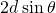. Constructive interference results when this distance is an integer multiple of the wavelength. This condition is captured by the Bragg equation,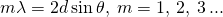where m is a positive integer and d is the spacing between the planes. Following the Law of Reflection, both the incident and reflected waves are described by the same angle,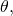but unlike the general practice in geometric optics,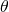is measured with respect to the surface itself, rather than the normal.

X-ray diffraction with a crystal. Two incident waves reflect off two planes of a crystal. The difference in path lengths is indicated by the dashed line.X-Ray Diffraction with Salt Crystals Common table salt is composed mainly of NaCl crystals. In a NaCl crystal, there is a family of planes 0.252 nm apart. If the first-order maximum is observed at an incidence angle of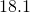, what is the wavelength of the X-ray scattering from this crystal?

Strategy Use the Bragg equation, (Figure),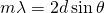, to solve for.

Solution For first-order,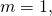and the plane spacing d is known. Solving the Bragg equation for wavelength yields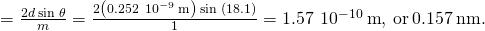Significance The determined wavelength fits within the X-ray region of the electromagnetic spectrum. Once again, the wave nature of light makes itself prominent when the wavelength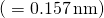is comparable to the size of the physical structures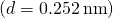it interacts with.

Check Your Understanding For the experiment described in (Figure), what are the two other angles where interference maxima may be observed? What limits the number of maxima?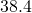and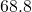; Between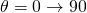, orders 1, 2, and 3, are all that exist.

Although (Figure) depicts a crystal as a two-dimensional array of scattering centers for simplicity, real crystals are structures in three dimensions. Scattering can occur simultaneously from different families of planes at different orientations and spacing patterns known as called Bragg planes, as shown in (Figure). The resulting interference pattern can be quite complex.

Because of the regularity that makes a crystal structure, one crystal can have many families of planes within its geometry, each one giving rise to X-ray diffraction.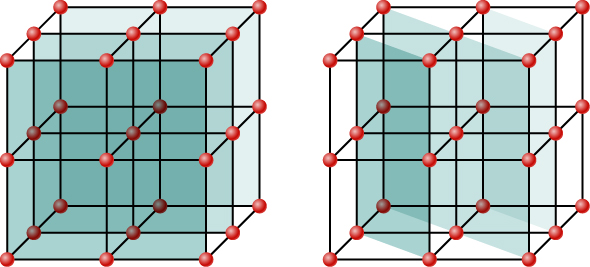### Summary

• X-rays are relatively short-wavelength EM radiation and can exhibit wave characteristics such as interference when interacting with correspondingly small objects.

### Conceptual Questions

Crystal lattices can be examined with X-rays but not UV. Why?

UV wavelengths are much larger than lattice spacings in crystals such that there is no diffraction. The Bragg equation implies a value for sin⁡θ greater than unity, which has no solution.

### Problems

X-rays of wavelength 0.103 nm reflects off a crystal and a second-order maximum is recorded at a Bragg angle of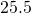. What is the spacing between the scattering planes in this crystal?

A first-order Bragg reflection maximum is observed when a monochromatic X-ray falls on a crystal at a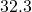angle to a reflecting plane. What is the wavelength of this X-ray?

0.120 nm

An X-ray scattering experiment is performed on a crystal whose atoms form planes separated by 0.440 nm. Using an X-ray source of wavelength 0.548 nm, what is the angle (with respect to the planes in question) at which the experimenter needs to illuminate the crystal in order to observe a first-order maximum?

The structure of the NaCl crystal forms reflecting planes 0.541 nm apart. What is the smallest angle, measured from these planes, at which X-ray diffraction can be observed, if X-rays of wavelength 0.085 nm are used?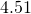On a certain crystal, a first-order X-ray diffraction maximum is observed at an angle of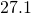relative to its surface, using an X-ray source of unknown wavelength. Additionally, when illuminated with a different, this time of known wavelength 0.137 nm, a second-order maximum is detected at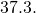Determine (a) the spacing between the reflecting planes, and (b) the unknown wavelength.

Calcite crystals contain scattering planes separated by 0.30 nm. What is the angular separation between first and second-order diffraction maxima when X-rays of 0.130 nm wavelength are used?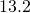The first-order Bragg angle for a certain crystal is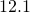. What is the second-order angle?

### Glossary

Bragg planes
families of planes within crystals that can give rise to X-ray diffraction
X-ray diffraction
technique that provides the detailed information about crystallographic structure of natural and manufactured materials